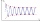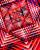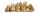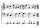# 6th grade (11y) + expression of a variable from the formula - math problems

1. Water tankWhat is the height of the cuboid-shaped tank with the bottom dimensions of 80 cm and 50 cm if the 480 liters of water reaches 10 cm below the top?
2. Isosceles triangleThe circumference of the isosceles triangle is 32.5 dm. Base length is 153 cm. How long is the leg of this triangle?
3. Two thirdsFind two-thirds of the number equal to two-thirds of 99
4. A cylindrical tankA cylindrical tank can hold 44 cubic meters of water. If the radius of the tank is 3.5 meters, how high is the tank?
5. Sum of four numbersThe sum of four consecutive natural numbers is 114. Find them.
6. Simple sequenceContinue with this series of numbers: 1792,448, 112, _, _
7. ExpressionSolve for a specified variable: P=a+4b+3c, for a
8. TwentyTwenty rabbits are put in 4 cells so that there are different number of rabbits in each cell contains at least 3 rabbits. What is the largest possible number of rabbits in one cell
9. A rectangleA rectangle has an area of 36 cm2. What could the length and width of rectangle be?
10. The perimeter 3The perimeter of a rectangle is 35 cm. The ratio of the length to its width is 3:2. Calculate the dimensions of the rectangle
11. Rectangle 45The perimeter of a rectangle is 60cm. If the length of the rectangle is 20cm. a)find the width b)find the area.
12. Number of songsWrite an expression for the number of songs they need for this show. Evan and Peter have a radio show that has 2 parts. They need 4 fewer than 11 songs in the first part. In the second part, they need 5 fewer than 3 times the number of songs in the first p
13. Steel tubeThe steel tube has an inner diameter of 4 cm and an outer diameter of 4.8 cm. The density of the steel is 7800 kg/m3. Calculate its length if it weighs 15 kg.

We apologize, but in this category are not a lot of examples.
Do you have an interesting mathematical word problem that you can't solve it? Enter it, and we can try to solve it.

To this e-mail address, we will reply solution; solved examples are also published here. Please enter the e-mail correctly and check whether you don't have a full mailbox.

Please do not submit problems from current active competitions such as Mathematical Olympiad, correspondence seminars etc...